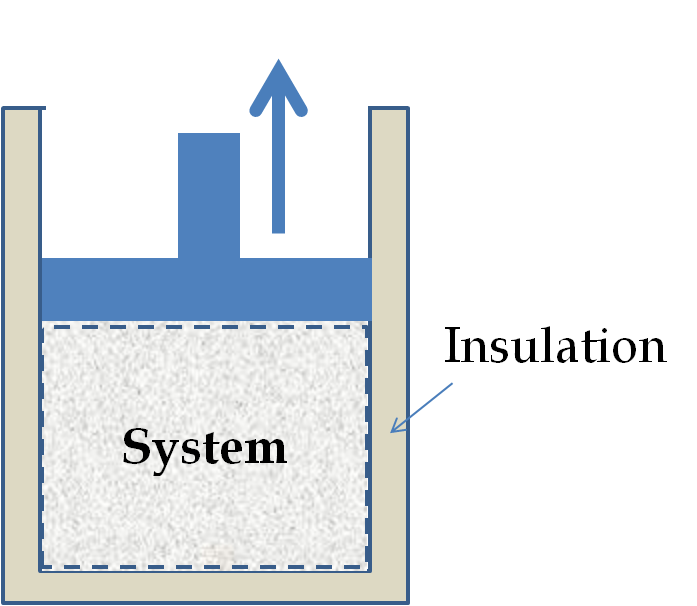## Section21.2Thermodynamic Processes

The thermodynamic state of a system can change as a result of interaction with the environment. For instance, you can change pressure, temperature, and/or volume of a gas by heating the gas. The state of the system may change gradually and in a well-defined way or change very abruptly. We call the process of change a thermodynamic process.

### Subsection21.2.1Quasi-static Processes

A quasi-static process refers to an idealized or imagined process where the change in state is made infinitesimally slowly so that at each instant the system can be assumed to be in a thermodynamic equilibrium within itself and with the environment.

For instance, imagine heating 1 kg of water from a temperature $20^{\circ}\text{C}$ to $21^{\circ}\text{C}$ at a constant pressure of $1\text{ atm}\text{.}$ To heat the water very slowly we may imagine placing the container with water in a large bath which can be slowly heated such that the temperature of the bath can rise infinitesimally slowly from $20^{\circ}\text{C}$ to $21^{\circ}\text{C}\text{.}$ If we put 1 kg water at $20^{\circ}\text{C}$ directly into a bath which is at $21^{\circ}\text{C}\text{,}$ the temperature of water will rise rapidly to $21^{\circ}\text{C}$ in a non-quasi-static way.

Quasi-static processes are done slowly enough that the system remains at thermodynamic equilibrium at each instant despite the fact that system changes over time. The thermodynamic equilibrium of the system is necessary for the system to have well-defined values of macroscopic properties such as temperature and pressure of the system at each instant of the process. Therefore, quasi-static processes can be shown as well-defined paths in state diagram of the system as shown in Figure 21.2.1. If a finite change occurs fast, we would not know the intermediate states between the initial and final states. Therefore, we display such non-quasistatic processes by dashed curves or lines as shown in the figure.

Since quasi-static process can be analyzed analytically, we will mostly study quasi-static processes in this book. We have already seen that in a quasi-static process, work by a gas is given by $pdV\text{.}$ Even heat can be written in analytic form if the quasi-static process is also reversible as we will see in the chapter on entropy.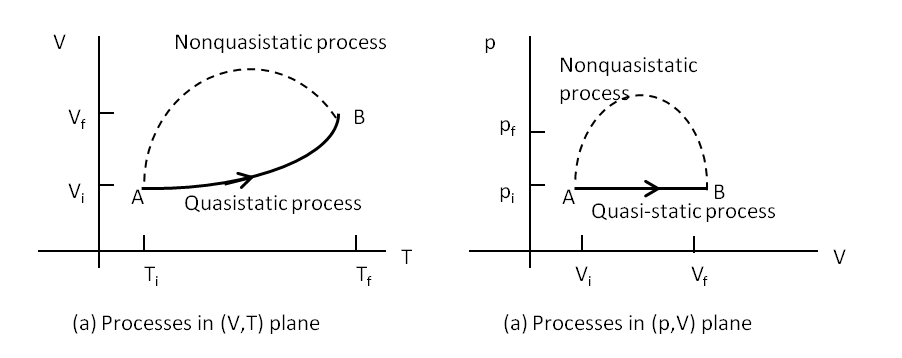Figure 21.2.1. Quasi-static and nonquasistatic processes between states A and B of a gas. In a quasi-static process the path of the process between A and B can be drawn in a state diagram since all the states the system goes through are known. In a nonquasistatic process the states between A and B are not known, and hence no path can be drawn. For a gas, you can draw a process in a plane of any pair of two state variables. In (a) the process is drawn in $(V,T)$ plane, and in (b) in the $(p,V)$ plane. At each point of the process, the pressure in the $(V,T)$ plane and temperature in the $(p,V)$ plane can be deduced by using the equation of state.

### Subsection21.2.2Isothermal Process

An isothermal process is a transformation during which temperature of the system is kept fixed. Say, you want to expand a gas at a constant temperature. You can place the gas in a heat-conductive container with a movable piston to heat flow and expansion. Then, you would place the container in a large bath, whose temperature you would maintain by heating or cooling it. You can vary the pressure in the gas by some weights on the piston as illustrated in Figure 21.2.2. Since the piston is freely movable, the pressure inside $P_{\text{in}}$ is balanced by the pressure outside $P_{\text{out}}$ by some weights on the piston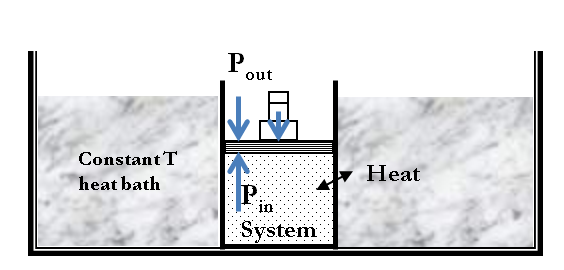Figure 21.2.2. Expanding a system at a constant temperature. Removing weights on the piston leads to an imbalance of forces on the piston which causes the piston to move up. As the piston moves up the temperature is lowered momentarily which cause heat to flow from the heat bath to the system. The energy to move the piston eventually comes from the heat bath.

As weights on the piston are removed, an imbalance of forces on the piston develops. The net non-zero force on the piston would cause the piston to accelerate, resulting in an increase in volume. The expansion of the gas cools the gas to a lower temperature, which makes it possible for the heat to enter from the heat bath into the system until the temperature of the gas is reset to the temperature of the heat bath.

If weights are removed in infinitesimal steps, the pressure in the system will decrease infinitesimally slowly. This way, an isothermal process can be conducted quasi-statically, which we can represent by a line in $(T,p)\text{,}$ $(T,V)$ or $(p,V)$ plane of the gas. On $(T,p)$ and $(T,V)$ diagrams a quasi-static isothermal process will be a straight line perpendicular to the temperature axis, while in the $(p,V)$ diagram, an isothermal is usually a curved line as shown in Figure 21.2.3. For an ideal gas an isothermal process is hyperbolic curve since $p\sim \frac{1}{V}$ for an ideal gas at constant temperature.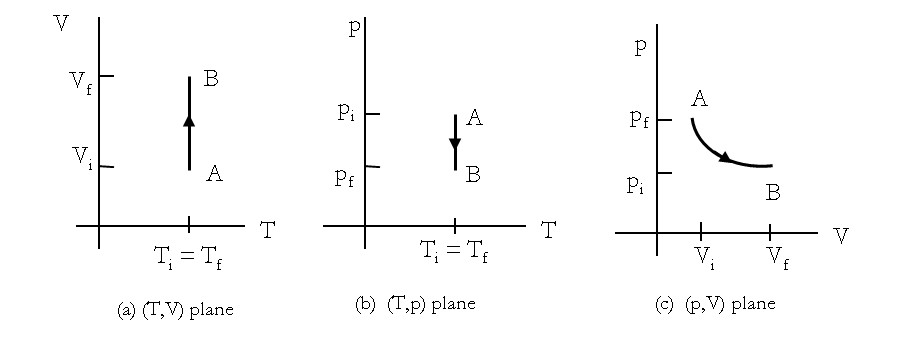Figure 21.2.3. An isothermal expansion from a state labeled A to another state labeled B in three planes of $(p,V,T)$ space.

An isothermal process is always conducted quasi-statically, since it to be isothermal throughout the change of volume, you must be able to state the temperature of the system at each step, which is possible only if the system is in thermal equilibrium continuously. Of course, the system must go out of equilibrium for the state to change, but for a quasi-static processes we imagine that the process is conducted in infinitesimal steps such that these departures from equilibrium can be made as brief and as small as we like.

In the $pV$-plane different temperatures correspond to different curves. They are called isotherms. In Figure 21.2.4, I have plotted isotherms at various temperatures for an ideal gas by using the ideal gas law, $pV = nRT = \text{constant}\text{,}$ for an isotherm. Different isotherms correspond to different values of $T\text{.}$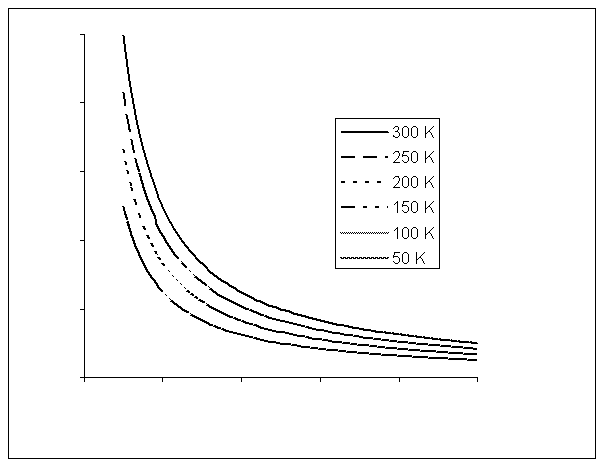Figure 21.2.4. Isotherms of one mole of an Ideal Gas. Each isotherm shows the states that can be reached by isothermal process from a point on the curve.

### Subsection21.2.3Isobaric and Isochoric Processes

Besides the isothermal processes, other quasi-static processes of interest for gases are isobaric and isochoric processes. An isobaric process is a process where the pressure of the system does not change while an isochoric process is a process where the volume of the system does not change.